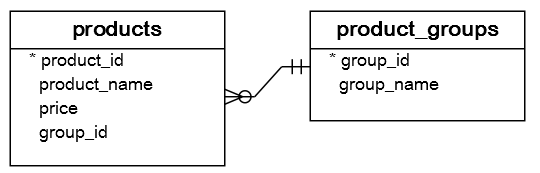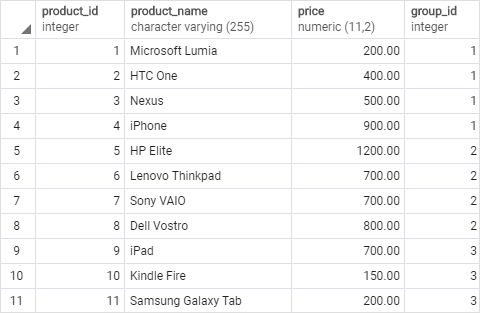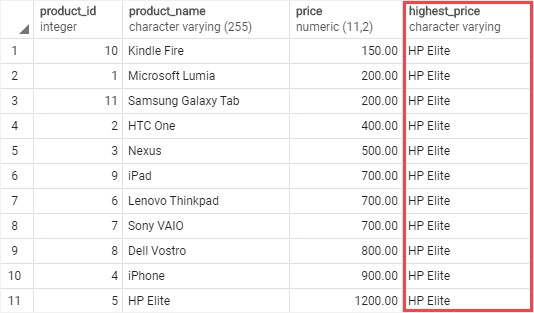# PostgreSQL Tutorial: LAST_VALUE Function

August 7, 2023

Summary: in this tutorial, you will learn how to get the last value in an ordered partition of a result set by using the PostgreSQL `LAST_VALUE()` function.

## Introduction to PostgreSQL `LAST_VALUE()` function

The `LAST_VALUE()` function returns the last value in an ordered partition of a result set.

The syntax of the `LAST_VALUE()` function is as follows:

``````LAST_VALUE ( expression )
OVER (
[PARTITION BY partition_expression, ... ]
ORDER BY sort_expression [ASC | DESC], ...
)
``````

In this syntax:

### `expression`

The `expression` can be an expression, column, or subquery evaluated against the value of the last row in an ordered partition of the result set.

The `expression` must return a single value. And it cannot be a window function.

### `PARTITION BY` clause

The `PARTITION BY` clause divides rows of the result set into partitions to which the `LAST_VALUE()` function is applied.

If you omit the `PARTITION BY` clause, the `LAST_VALUE()` function will treat the whole result set as a single partition.

### `ORDER BY` clause

The `ORDER BY` clause specifies the sort order for rows in each partition to which the `LAST_VALUE()` function is applied.

### `frame_clause`

The `frame_clause` defines the subset of rows in the current partition to which the `LAST_VALUE()` function is applied.

## PostgreSQL `LAST_VALUE()` function examples

We will use the `products` table created in the window function tutorial for the demonstration:Here are the contents of the data of the `products` table:### 1) Using PostgreSQL `LAST_VALUE()` over a result set example

The following example uses the `LAST_VALUE()` function to return all products together with the product that has the highest price:

``````SELECT
product_id,
product_name,
price,
LAST_VALUE(product_name)
OVER(
ORDER BY price
RANGE BETWEEN
UNBOUNDED PRECEDING AND
UNBOUNDED FOLLOWING
) highest_price
FROM
products;
``````In this example:

• We skipped the `PARTITION BY` clause in the `LAST_VALUE()` function, therefore, the `LAST_VALUE()` function treated the whole result set as a single partition.
• The `ORDER BY` clause sorted products by prices from low to high.
• The `LAST_VALUE()` picked the product name of the last row in the result set.

### 2) Using PostgreSQL `LAST_VALUE()` over a partition example

The following example uses the `LAST_VALUE()` function to return all products together with the most expensive product per product group:

``````SELECT
product_id,
product_name,
group_id,
price,
LAST_VALUE(product_name)
OVER(
PARTITION BY group_id
ORDER BY price
RANGE BETWEEN
UNBOUNDED PRECEDING AND
UNBOUNDED FOLLOWING
) highest_price
FROM
products;
``````In this example:

• The `PARTITION BY` clause divided rows by group id into three partitions specified by group id 1, 2, and 3.
• The `ORDER BY` clause sorted products in each product group ( or partition) from low to high.
• The `RANGE BETWEEN UNBOUNDED PRECEDING AND UNBOUNDED FOLLOWING` clause defined the frame starting from the first row and ending at the last row of each partition.
• The `LAST_VALUE()` function was applied to each partition separately and returned the product name of the last row in each partition.

In this tutorial, you have learned how to return the last value in an ordered partition of a result set by using the PostgreSQL `LAST_VALUE()` window function.